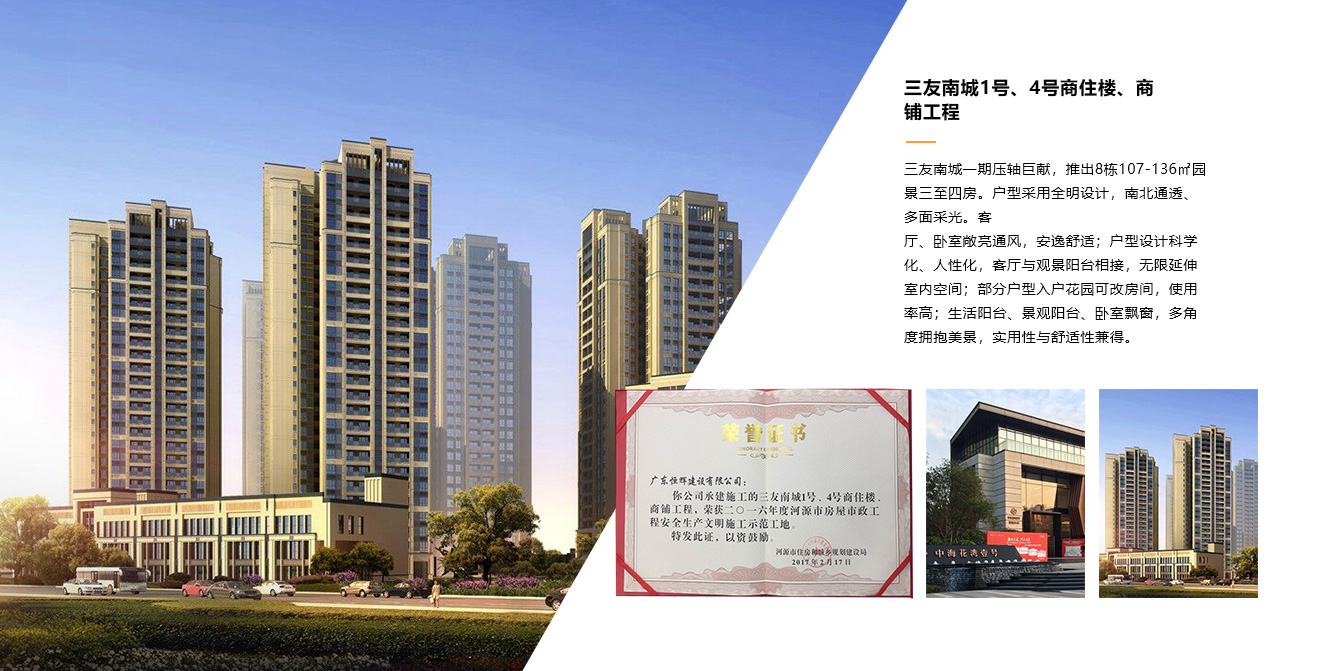TOP

# TYPICALPROJECT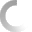• ×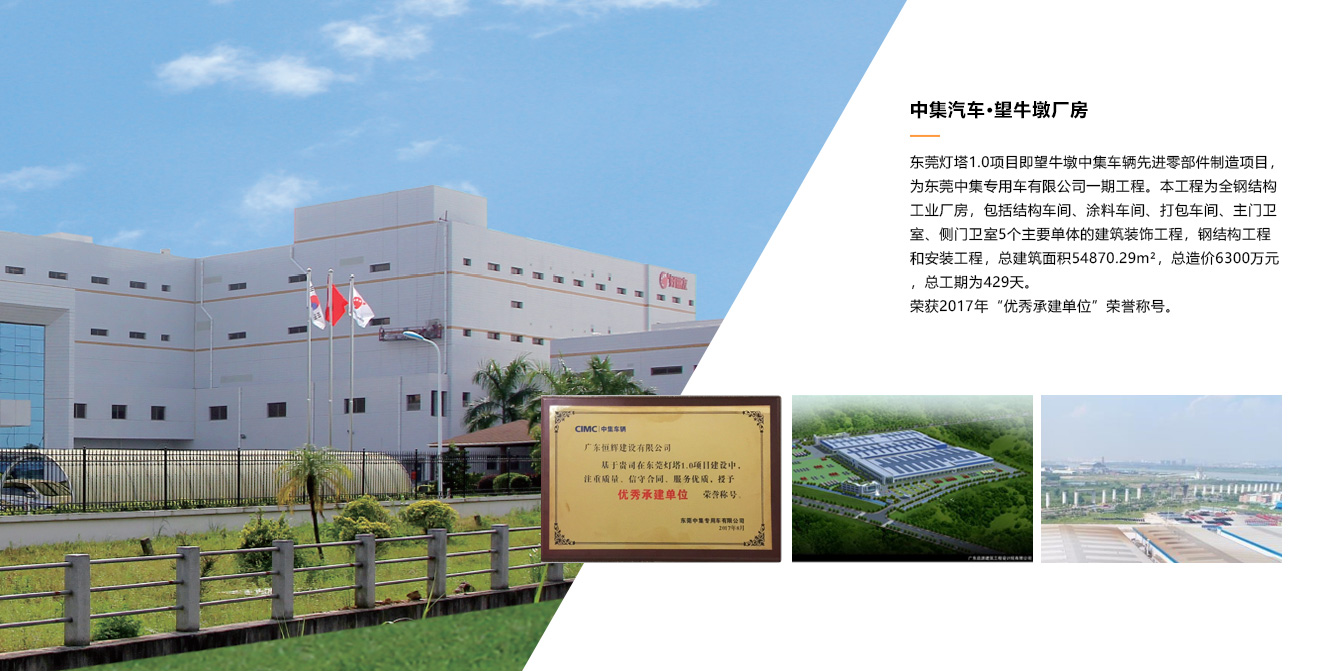• ×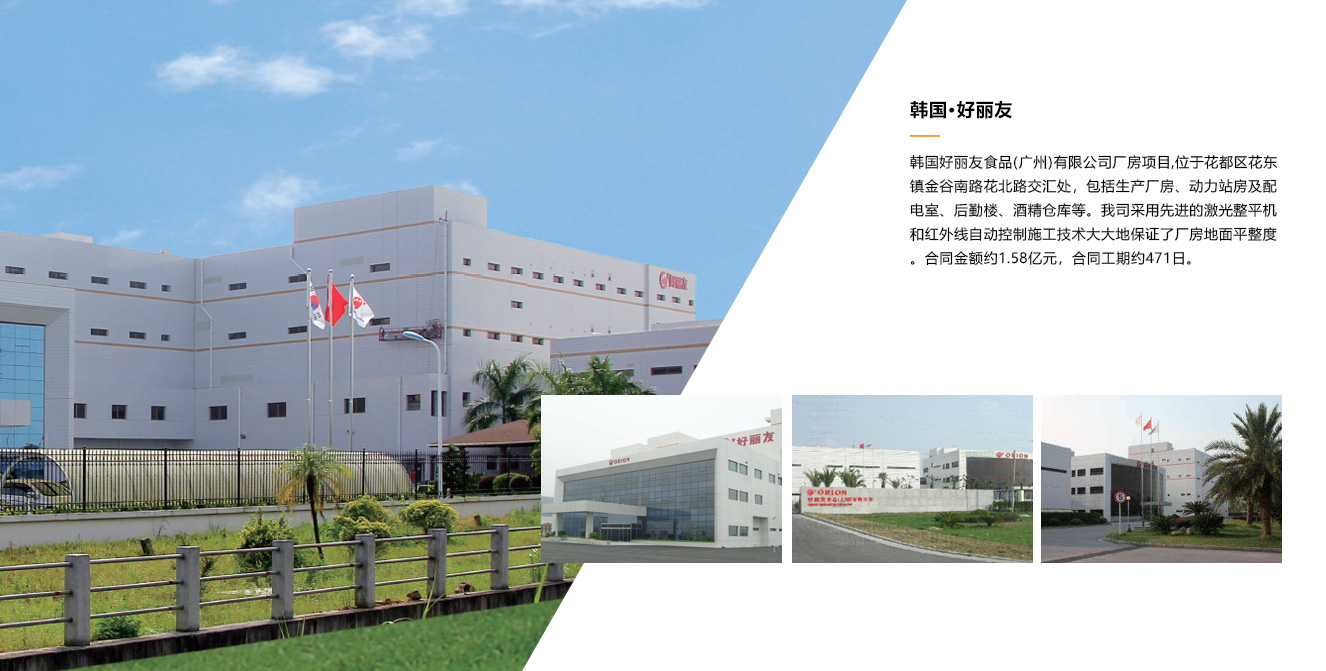• ×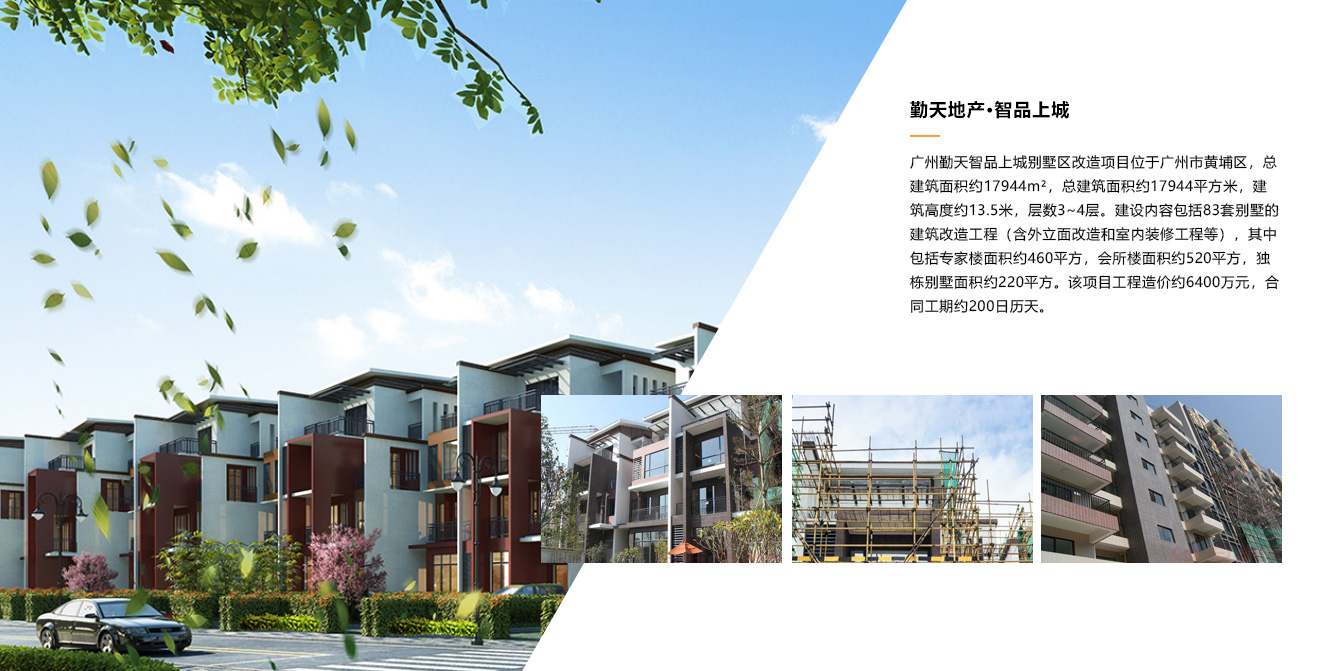• ×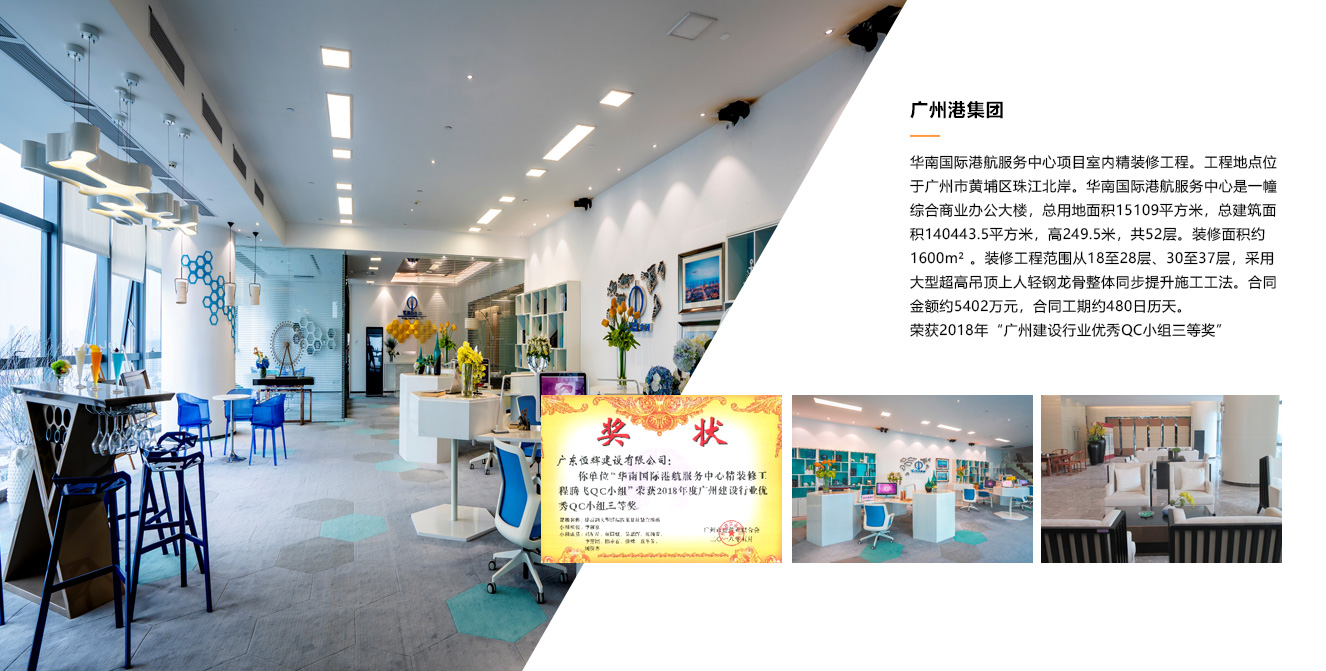• ×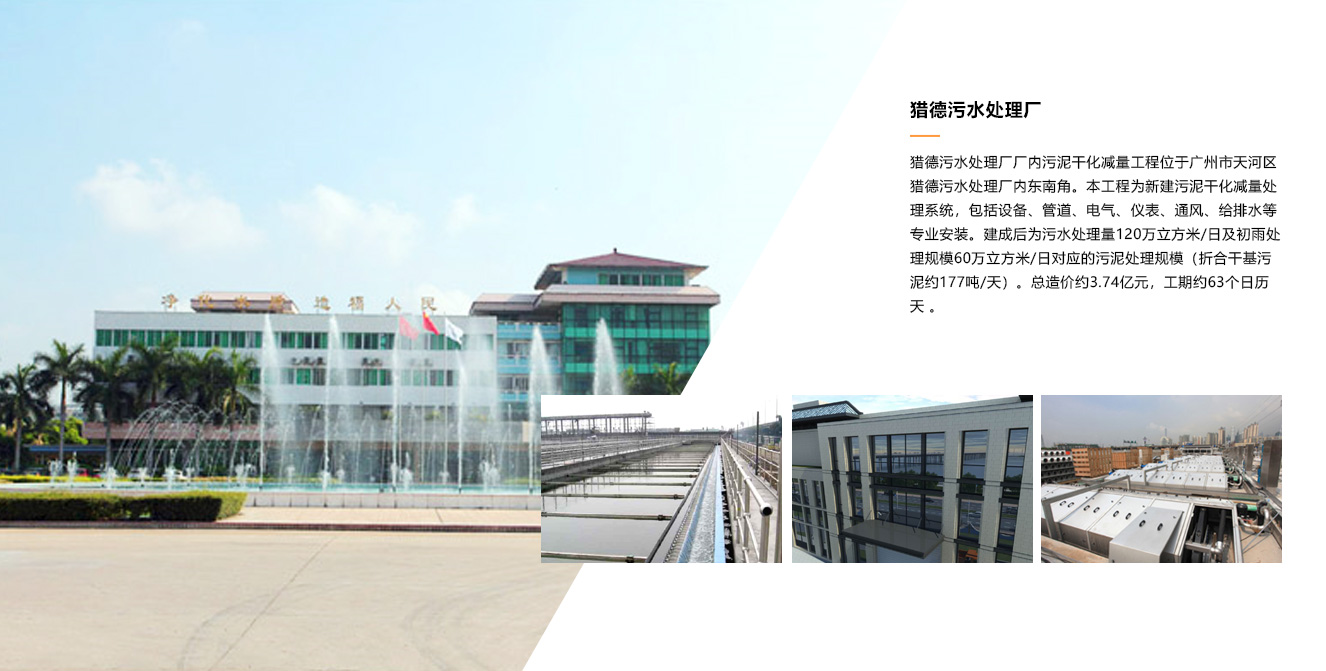• ×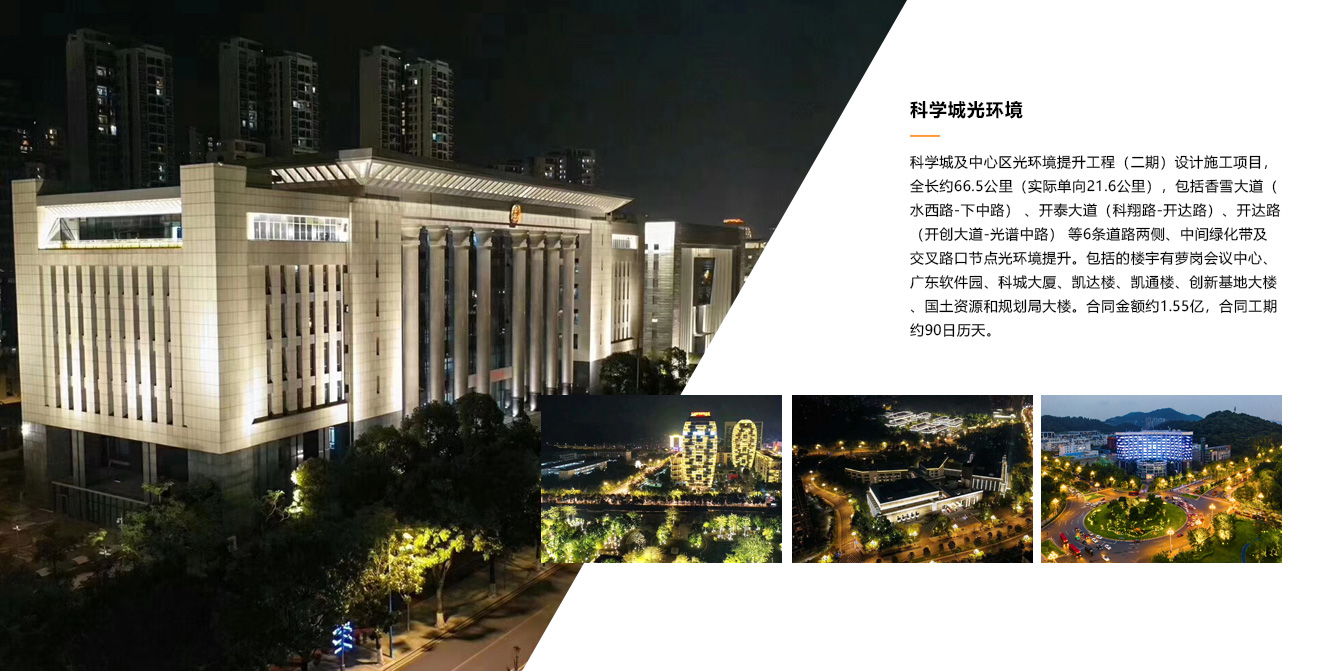• ×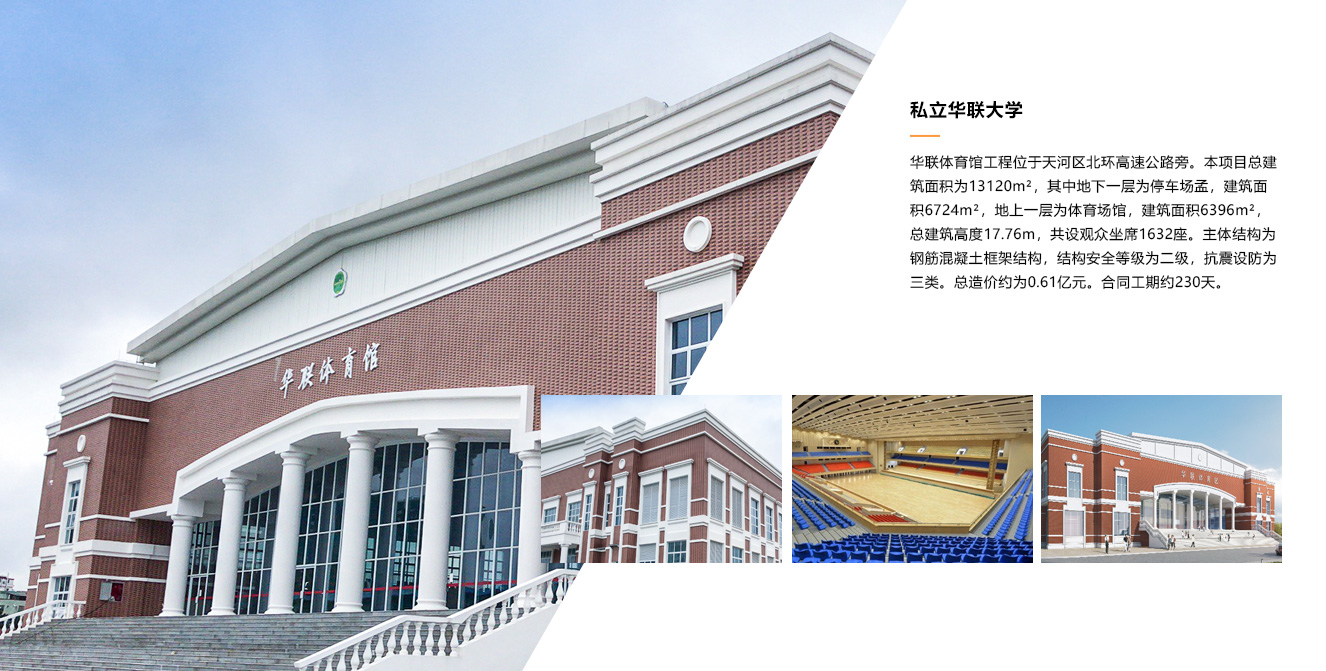• ×• ×• ×• ×• ×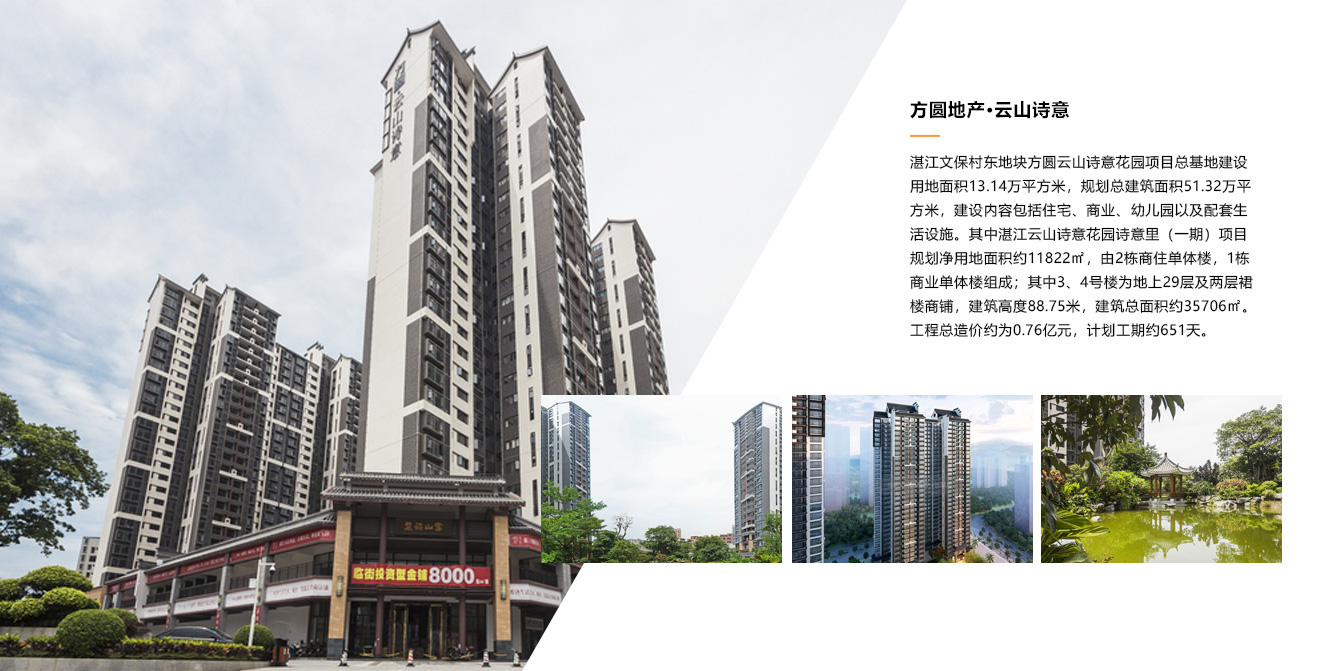• ×• ×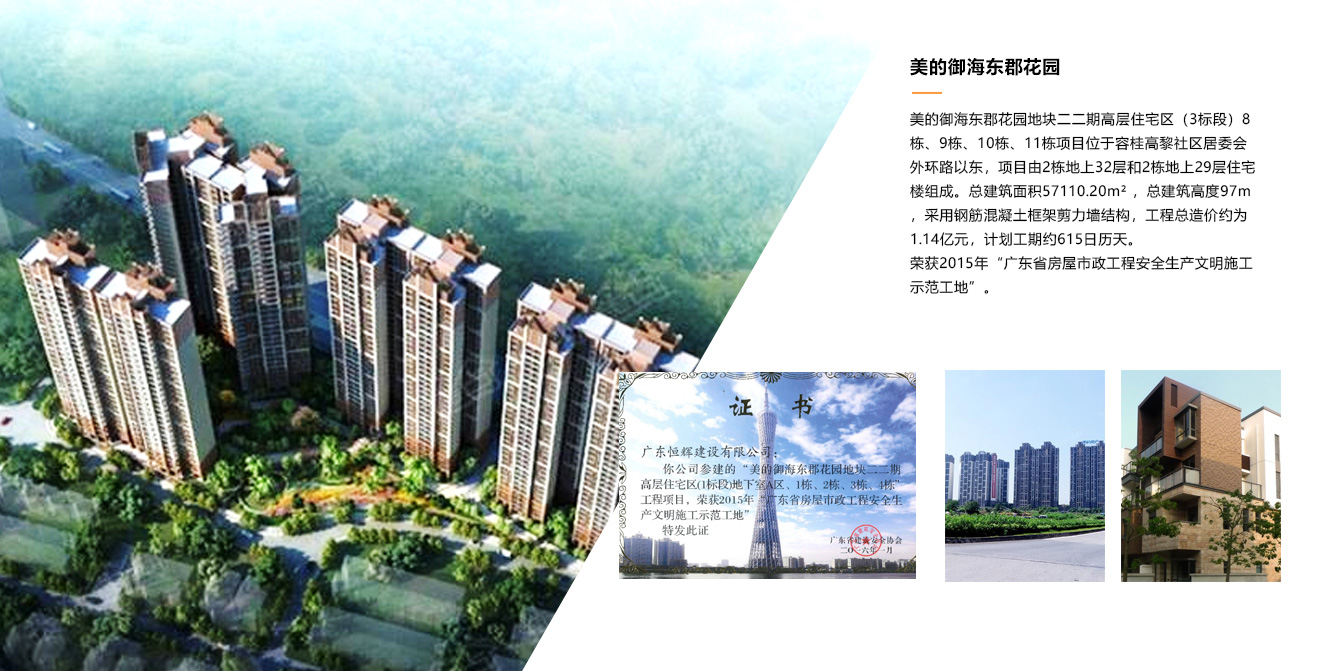• ×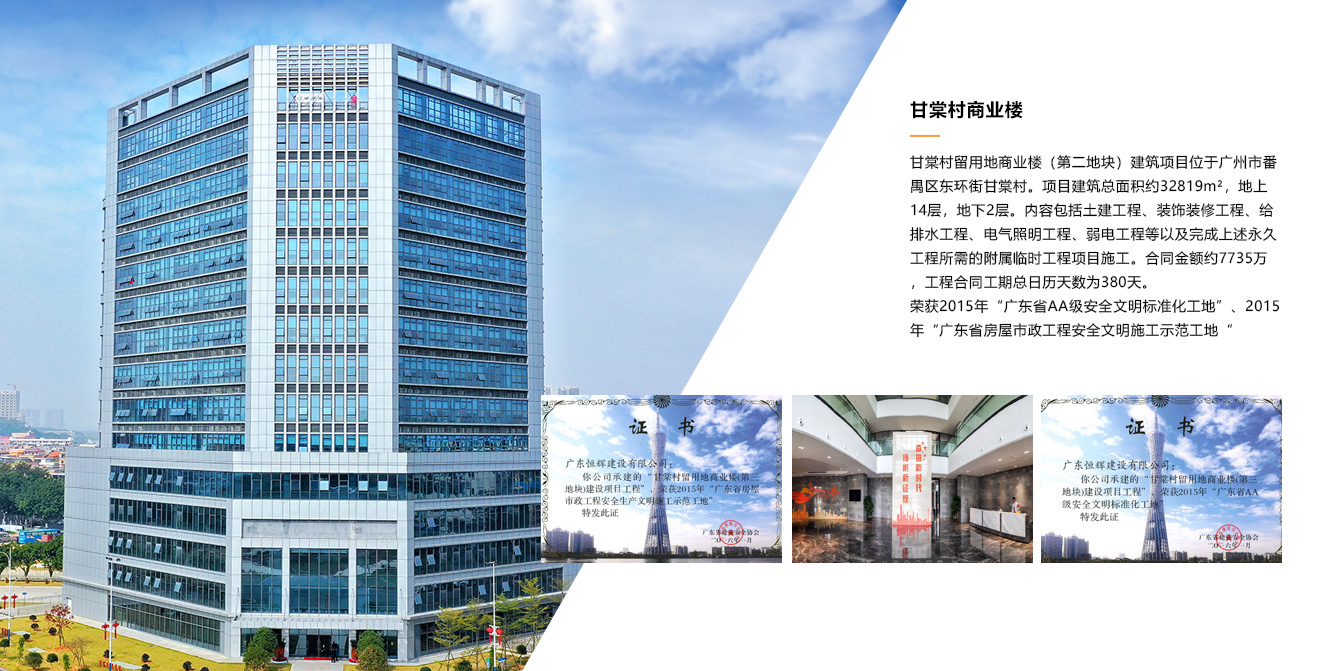• ×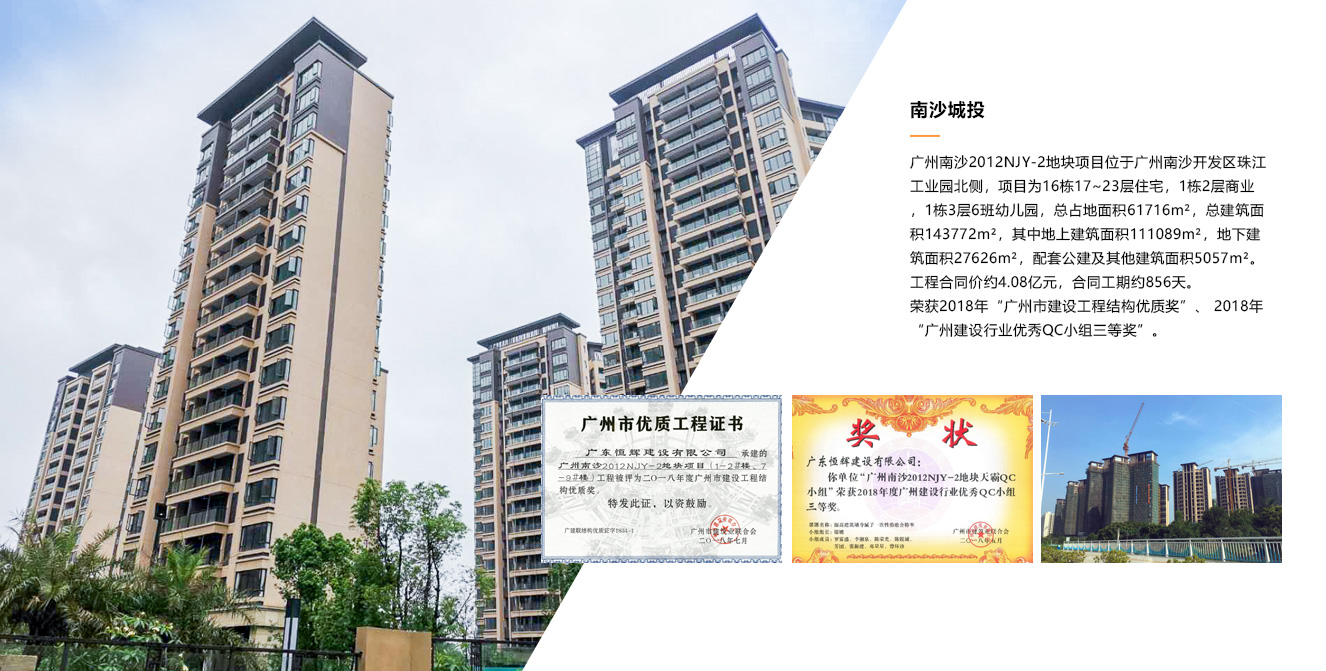• ×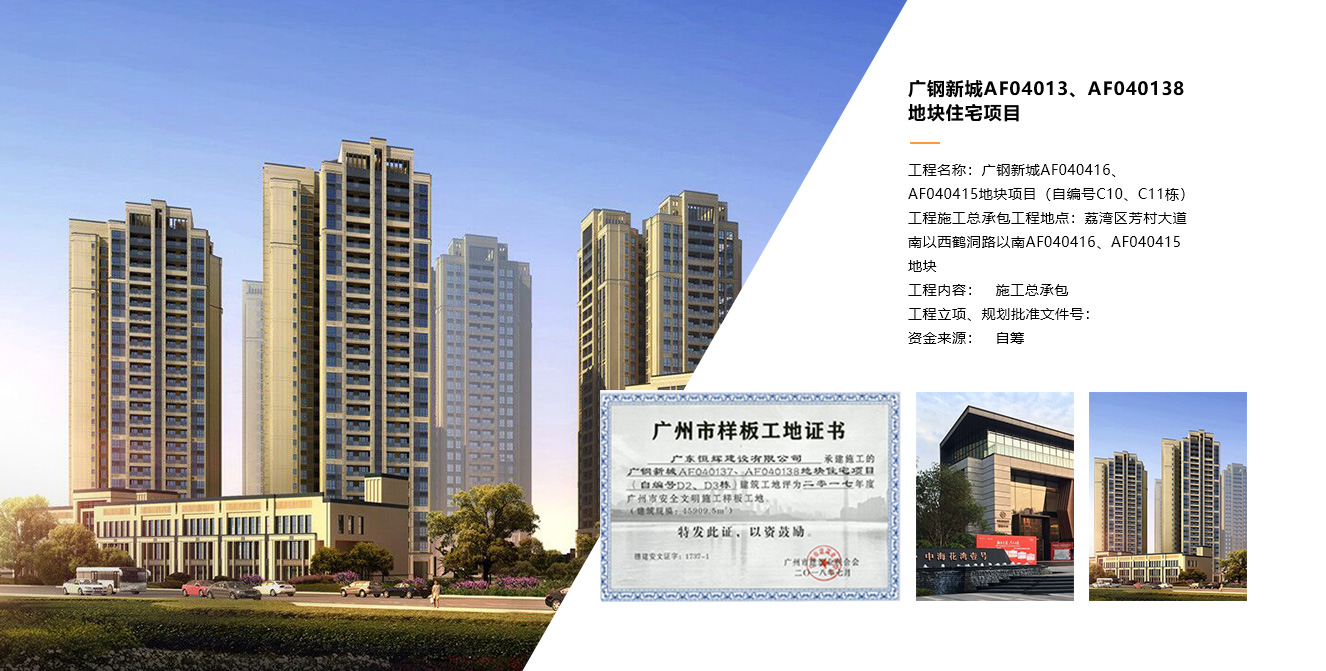• ×